Next: Vector Algebra Up: Vector Algebra and Vector Previous: Introduction

Scalars and Vectors

Many physical quantities (e.g., mass, energy) are entirely defined by a numerical magnitude (in appropriate units). Such quantities, which have no directional element, are known as scalars. Moreover, since scalars can be represented by real numbers, it follows that they obey the familiar laws of ordinary algebra. However, there exits a second class of physical quantities (e.g., velocity, acceleration, force) which are only completely defined when both a numerical magnitude and a direction in space is specified. Such quantities are known as vectors. By definition, a vector obeys the same algebra as a displacement in space, and may thus be represented geometrically by a straight-line,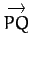(say), where the arrow indicates the direction of the displacement (i.e., from pointto point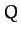)--see Figure A.96. The magnitude of the vector is represented by the length of the straight-line.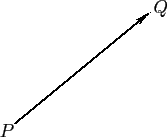It is conventional to denote vectors by bold-faced symbols (e.g.,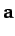,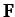) and scalars by non-bold-faced symbols (e.g.,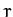,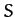). The magnitude of a general vector,, is denoted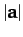, or just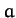, and is, by definition, always greater than or equal to zero. It is convenient to define a vector with zero magnitude--this is denoted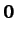, and has no direction. Finally, two vectors,and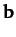, are said to be equal when their magnitudes and directions are identical.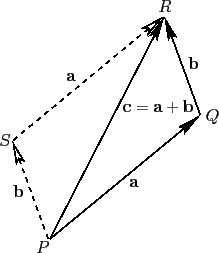Next: Vector Algebra Up: Vector Algebra and Vector Previous: Introduction
Richard Fitzpatrick 2011-03-31